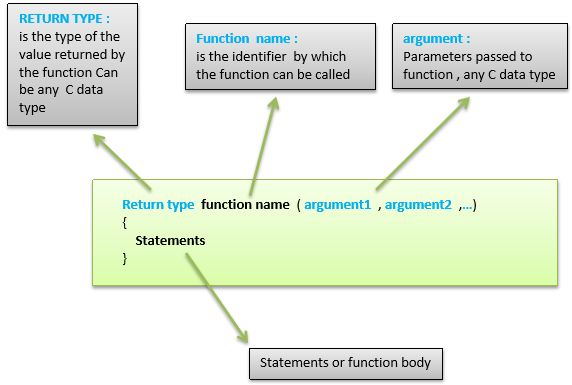# Arduino - 函数

• 函数可以帮助程序员保持井井有条。通常这有助于概念化程序。

• 函数在一个地方编码一个操作，因此只需要考虑和调试一次该函数。

• 如果需要更改代码，这也减少了修改错误的机会。

• 函数使整个程序更小，更紧凑，因为代码段可以重复使用多次。

• 通过使代码模块化，可以更容易地在其他程序中重用代码，并且使用函数通常会使代码更具可读性。

Arduino 草图或程序中有两个必需的函数，即 setup()loop()。必须在这两个函数的括号外创建其他函数。## 函数声明

• 函数返回类型
• 函数名称
• 函数参数类型，无需编写参数名称

### 例

int sum_func (int x, int y) // function declaration {
int z = 0;
z = x+y ;
return z; // return the value
}

void setup () {
Statements // group of statements
}

Void loop () {
int result = 0 ;
result = Sum_func (5,6) ; // function call
}


• 函数返回类型
• 函数名称
• 函数参数类型，这里必须添加参数名称
• 函数体（调用函数时执行函数内的语句）

### 例

int sum_func (int , int ) ; // function prototype

void setup () {
Statements // group of statements
}

Void loop () {
int result = 0 ;
result = Sum_func (5,6) ; // function call
}

int sum_func (int x, int y) // function declaration {
int z = 0;
z = x+y ;
return z; // return the value
}